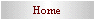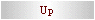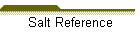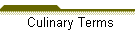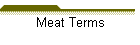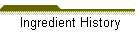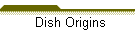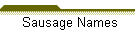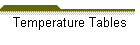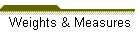METRIC CONVERSIONS These are the basic formulas used to convert recipe measurements from metric to US standard or from US standard to metric. These are not the only formulas that can be used but they may be the simplest. The metric system is a decimal based system. It is used through out the world and Canada. France adopted the metric system as early as 1799 AD. The US system is based on a much older unit of measurement from England. DRY WEIGHT: US to metric # of ounces x 28.35 = # of grams Metric to US # of grams 28.35 = # of ounces LIQUID MEASUREMENT: US to metric # of ounces x 30 = # of milliliters Metric to US # of milliliters 30 = # of ounces Other necessary equivalencies: US 3t = 1T 1 T = .5 oz 1 c = 8 oz 2 c = 1 pt 2 pt = 1 qt 4 qt = 1 gal 16 oz = 1 # METRIC: 1000 ml = 1L 1000g = 1 KG 100 cl = 1 L 10 dl = 1 L The metric system used prefixes form the Greek language. They are as follows: deca =10 hecto = 100 kilo = 1000 For less than 1: deci = .1 centi = .01 milli = .001 ENGLISH TO METRIC METRIC TO ENGLISH 1 inch = 2.54 centimeters (cm) 1 cm = 0.3937 inch 1 foot = 30.5 centimeters (cm) 1 cm = 0.0328 feet 1 yard = 0.914 meters (m) 1 m = 1.094 yards / 39.37 inches 1 mile = 1.609 kilometers (km) 1 km = 0.6214 mile quart = 0.9464 liter (l) 1L = 1.06 quarts 1 ounce = 28.35 grams (gm) 1 gm = 0.0353 ounce 1 pound = 0.4536 kilograms (kg) 1 kg = 2.2 pounds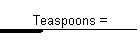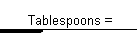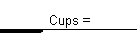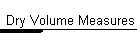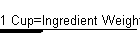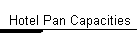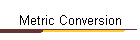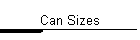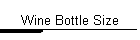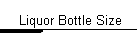.